Publicité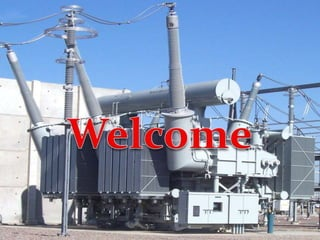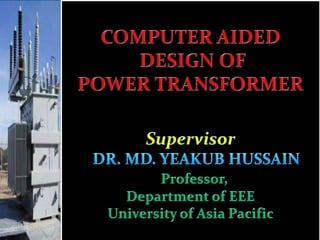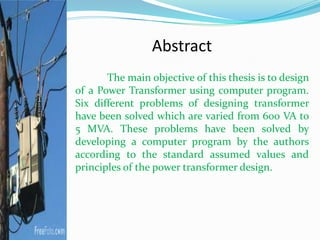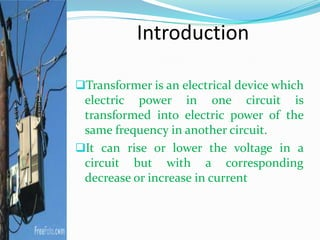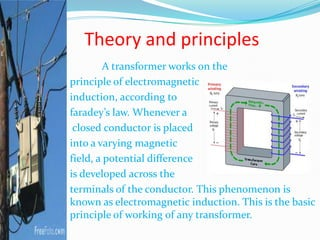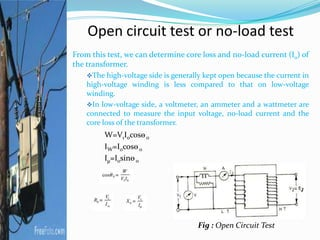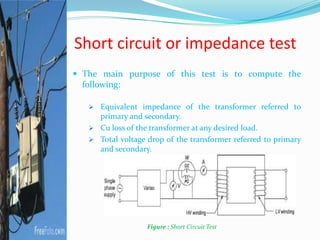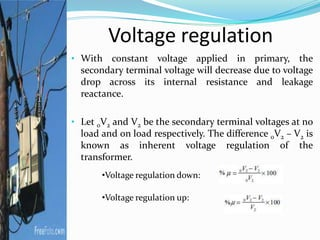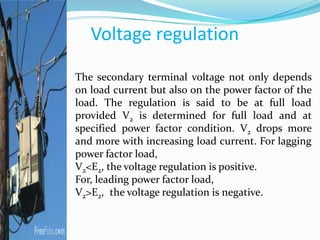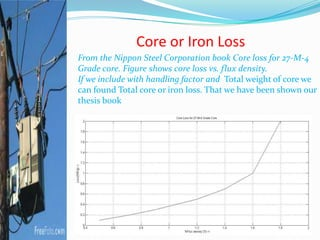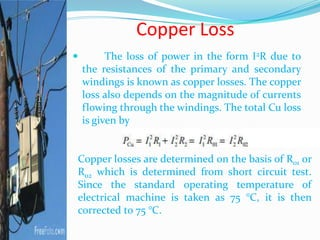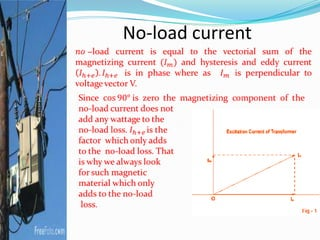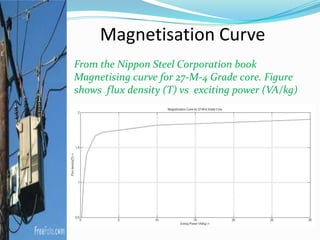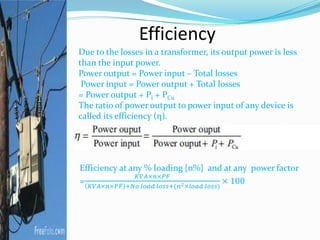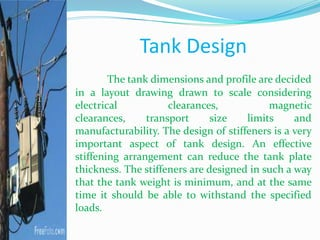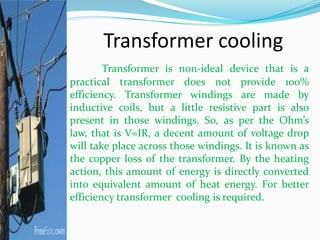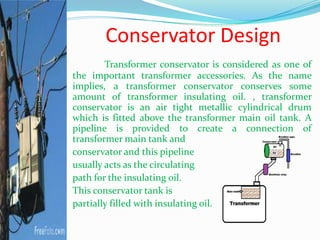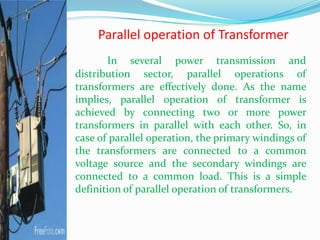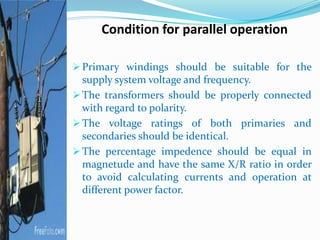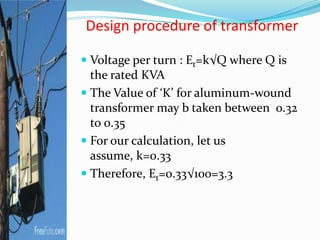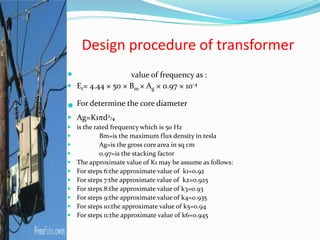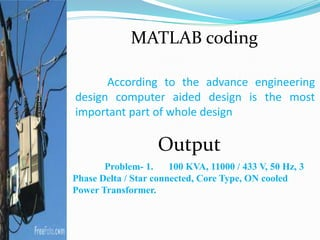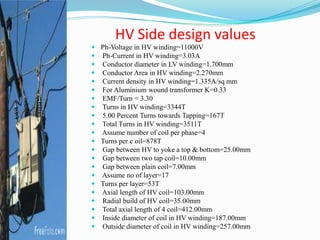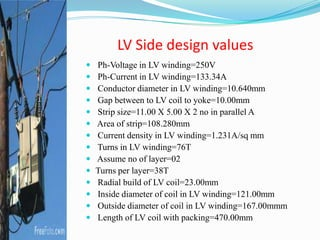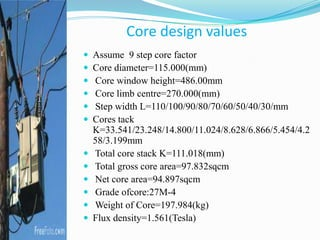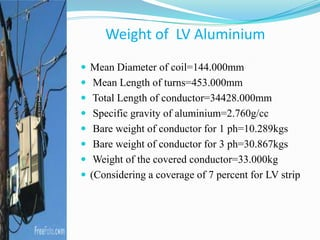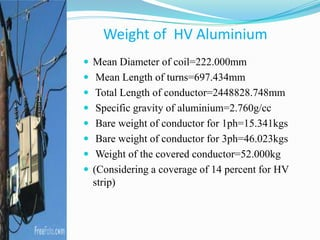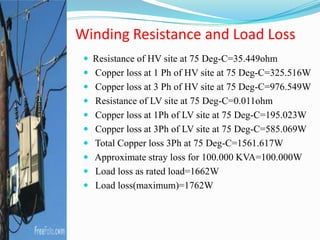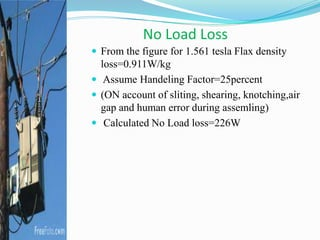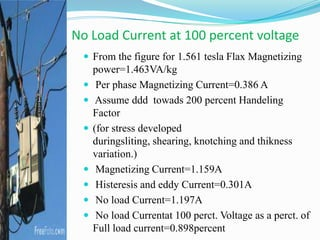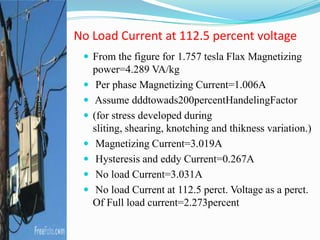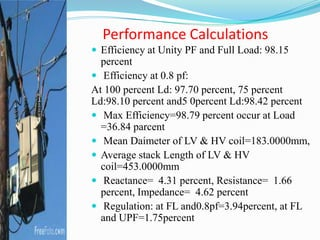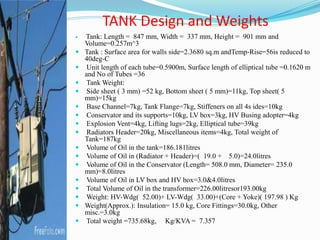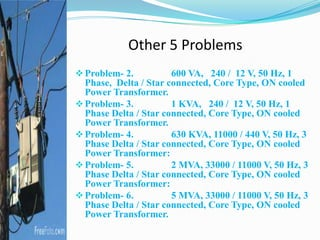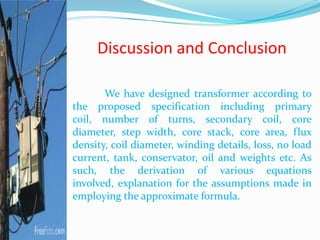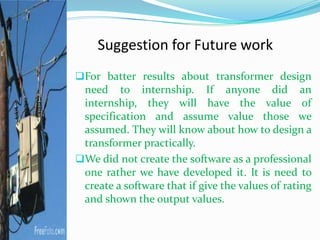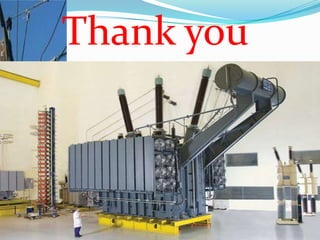1 sur 38
Publicité

### Presentation Design of Computer aided design of power transformer

1. Our group members:  Mizanur Rahman khan Reg:09208025  Md. Soriful Islam Rasel Reg:09208008  Md. Toukir Ahmed Reg:09208005  M.K. Masud Hasan Reg:09208031
2. Abstract The main objective of this thesis is to design of a Power Transformer using computer program. Six different problems of designing transformer have been solved which are varied from 600 VA to 5 MVA. These problems have been solved by developing a computer program by the authors according to the standard assumed values and principles of the power transformer design.
3. Introduction Transformer is an electrical device which electric power in one circuit is transformed into electric power of the same frequency in another circuit. It can rise or lower the voltage in a circuit but with a corresponding decrease or increase in current
4. Theory and principles A transformer works on the principle of electromagnetic induction, according to faradey’s law. Whenever a closed conductor is placed into a varying magnetic field, a potential difference is developed across the terminals of the conductor. This phenomenon is known as electromagnetic induction. This is the basic principle of working of any transformer.
5. Open circuit test or no-load test From this test, we can determine core loss and no-load current (I0) of the transformer. The high-voltage side is generally kept open because the current in high-voltage winding is less compared to that on low-voltage winding. In low-voltage side, a voltmeter, an ammeter and a wattmeter are connected to measure the input voltage, no-load current and the core loss of the transformer. W=V1I0cosѳ0 IW=I0cosѳ0 Iµ=I0sinѳ0 Fig : Open Circuit Test
6. Short circuit or impedance test  The main purpose of this test is to compute the following:  Equivalent impedance of the transformer referred to primary and secondary.  Cu loss of the transformer at any desired load.  Total voltage drop of the transformer referred to primary and secondary. Figure : Short Circuit Test
7. Voltage regulation • With constant voltage applied in primary, the secondary terminal voltage will decrease due to voltage drop across its internal resistance and leakage reactance. • Let 0V2 and V2 be the secondary terminal voltages at no load and on load respectively. The difference 0V2 – V2 is known as inherent voltage regulation of the transformer. •Voltage regulation down: •Voltage regulation up:
8. Voltage regulation The secondary terminal voltage not only depends on load current but also on the power factor of the load. The regulation is said to be at full load provided V2 is determined for full load and at specified power factor condition. V2 drops more and more with increasing load current. For lagging power factor load, V2<E2, the voltage regulation is positive. For, leading power factor load, V2>E2, the voltage regulation is negative.
9. Core or Iron Loss From the Nippon Steel Corporation book Core loss for 27-M-4 Grade core. Figure shows core loss vs. flux density. If we include with handling factor and Total weight of core we can found Total core or iron loss. That we have been shown our thesis book
10. Copper Loss  The loss of power in the form I2R due to the resistances of the primary and secondary windings is known as copper losses. The copper loss also depends on the magnitude of currents flowing through the windings. The total Cu loss is given by Copper losses are determined on the basis of R01 or R02 which is determined from short circuit test. Since the standard operating temperature of electrical machine is taken as 75 °C, it is then corrected to 75 °C.
12. Magnetisation Curve From the Nippon Steel Corporation book Magnetising curve for 27-M-4 Grade core. Figure shows flux density (T) vs exciting power (VA/kg)
13. Efficiency Due to the losses in a transformer, its output power is less than the input power. Power output = Power input – Total losses Power input = Power output + Total losses = Power output + Pi + PCu The ratio of power output to power input of any device is called its efficiency (η).
14. Tank Design The tank dimensions and profile are decided in a layout drawing drawn to scale considering electrical clearances, magnetic clearances, transport size limits and manufacturability. The design of stiffeners is a very important aspect of tank design. An effective stiffening arrangement can reduce the tank plate thickness. The stiffeners are designed in such a way that the tank weight is minimum, and at the same time it should be able to withstand the specified loads.
15. Transformer cooling Transformer is non-ideal device that is a practical transformer does not provide 100% efficiency. Transformer windings are made by inductive coils, but a little resistive part is also present in those windings. So, as per the Ohm’s law, that is V=IR, a decent amount of voltage drop will take place across those windings. It is known as the copper loss of the transformer. By the heating action, this amount of energy is directly converted into equivalent amount of heat energy. For better efficiency transformer cooling is required.
16. Conservator Design Transformer conservator is considered as one of the important transformer accessories. As the name implies, a transformer conservator conserves some amount of transformer insulating oil. , transformer conservator is an air tight metallic cylindrical drum which is fitted above the transformer main oil tank. A pipeline is provided to create a connection of transformer main tank and conservator and this pipeline usually acts as the circulating path for the insulating oil. This conservator tank is partially filled with insulating oil.
17. Parallel operation of Transformer In several power transmission and distribution sector, parallel operations of transformers are effectively done. As the name implies, parallel operation of transformer is achieved by connecting two or more power transformers in parallel with each other. So, in case of parallel operation, the primary windings of the transformers are connected to a common voltage source and the secondary windings are connected to a common load. This is a simple definition of parallel operation of transformers.
18. Condition for parallel operation  Primary windings should be suitable for the supply system voltage and frequency.  The transformers should be properly connected with regard to polarity.  The voltage ratings of both primaries and secondaries should be identical.  The percentage impedence should be equal in magnetude and have the same X/R ratio in order to avoid calculating currents and operation at different power factor.
19. Design procedure of transformer  Voltage per turn : Et=k√Q where Q is the rated KVA  The Value of ‘K’ for aluminum-wound transformer may b taken between 0.32 to 0.35  For our calculation, let us assume, k=0.33  Therefore, Et=0.33√100=3.3
20. Design procedure of transformer  value of frequency as :  Et= 4.44 × 50 × Bm × Ag × 0.97 × 10-4  For determine the core diameter  Ag=K1πd2/4  is the rated frequency which is 50 Hz  Bm=is the maximum flux density in tesla  Ag=is the gross core area in sq cm  0.97=is the stacking factor  The approximate value of K1 may be assume as follows:  For steps 6:the approximate value of k1=0.92  For steps 7:the approximate value of k2=0.925  For steps 8:the approximate value of k3=0.93  For steps 9:the approximate value of k4=0.935  For steps 10:the approximate value of k5=0.94  For steps 11:the approximate value of k6=0.945
21. According to the advance engineering design computer aided design is the most important part of whole design Problem- 1. 100 KVA, 11000 / 433 V, 50 Hz, 3 Phase Delta / Star connected, Core Type, ON cooled Power Transformer. Output MATLAB coding
22. HV Side design values  Ph-Voltage in HV winding=11000V  Ph-Current in HV winding=3.03A  Conductor diameter in LV winding=1.700mm  Conductor Area in HV winding=2.270mm  Current density in HV winding=1.335A/sq mm  For Aluminium wound transformer K=0.33  EMF/Turn = 3.30  Turns in HV winding=3344T  5.00 Percent Turns towards Tapping=167T  Total Turns in HV winding=3511T  Assume number of coil per phase=4  Turns per c oil=878T  Gap between HV to yoke a top & bottom=25.00mm  Gap between two tap coil=10.00mm  Gap between plain coil=7.00mm  Assume no of layer=17  Turns per layer=53T  Axial length of HV coil=103.00mm  Radial build of HV coil=35.00mm  Total axial length of 4 coil=412.00mm  Inside diameter of coil in HV winding=187.00mm  Outside diameter of coil in HV winding=257.00mm
23. LV Side design values  Ph-Voltage in LV winding=250V  Ph-Current in LV winding=133.34A  Conductor diameter in LV winding=10.640mm  Gap between to LV coil to yoke=10.00mm  Strip size=11.00 X 5.00 X 2 no in parallel A  Area of strip=108.280mm  Current density in LV winding=1.231A/sq mm  Turns in LV winding=76T  Assume no of layer=02  Turns per layer=38T  Radial build of LV coil=23.00mm  Inside diameter of coil in LV winding=121.00mm  Outside diameter of coil in LV winding=167.00mmm  Length of LV coil with packing=470.00mm
24. Core design values  Assume 9 step core factor  Core diameter=115.000(mm)  Core window height=486.00mm  Core limb centre=270.000(mm)  Step width L=110/100/90/80/70/60/50/40/30/mm  Cores tack K=33.541/23.248/14.800/11.024/8.628/6.866/5.454/4.2 58/3.199mm  Total core stack K=111.018(mm)  Total gross core area=97.832sqcm  Net core area=94.897sqcm  Grade ofcore:27M-4  Weight of Core=197.984(kg)  Flux density=1.561(Tesla)
25. Weight of LV Aluminium  Mean Diameter of coil=144.000mm  Mean Length of turns=453.000mm  Total Length of conductor=34428.000mm  Specific gravity of aluminium=2.760g/cc  Bare weight of conductor for 1 ph=10.289kgs  Bare weight of conductor for 3 ph=30.867kgs  Weight of the covered conductor=33.000kg  (Considering a coverage of 7 percent for LV strip
26.  Mean Diameter of coil=222.000mm  Mean Length of turns=697.434mm  Total Length of conductor=2448828.748mm  Specific gravity of aluminium=2.760g/cc  Bare weight of conductor for 1ph=15.341kgs  Bare weight of conductor for 3ph=46.023kgs  Weight of the covered conductor=52.000kg  (Considering a coverage of 14 percent for HV strip) Weight of HV Aluminium
27.  Resistance of HV site at 75 Deg-C=35.449ohm  Copper loss at 1 Ph of HV site at 75 Deg-C=325.516W  Copper loss at 3 Ph of HV site at 75 Deg-C=976.549W  Resistance of LV site at 75 Deg-C=0.011ohm  Copper loss at 1Ph of LV site at 75 Deg-C=195.023W  Copper loss at 3Ph of LV site at 75 Deg-C=585.069W  Total Copper loss 3Ph at 75 Deg-C=1561.617W  Approximate stray loss for 100.000 KVA=100.000W  Load loss as rated load=1662W  Load loss(maximum)=1762W Winding Resistance and Load Loss
28. No Load Loss  From the figure for 1.561 tesla Flax density loss=0.911W/kg  Assume Handeling Factor=25percent  (ON account of sliting, shearing, knotching,air gap and human error during assemling)  Calculated No Load loss=226W
29.  From the figure for 1.561 tesla Flax Magnetizing power=1.463VA/kg  Per phase Magnetizing Current=0.386 A  Assume ddd towads 200 percent Handeling Factor  (for stress developed duringsliting, shearing, knotching and thikness variation.)  Magnetizing Current=1.159A  Histeresis and eddy Current=0.301A  No load Current=1.197A  No load Currentat 100 perct. Voltage as a perct. of Full load current=0.898percent No Load Current at 100 percent voltage
30.  From the figure for 1.757 tesla Flax Magnetizing power=4.289 VA/kg  Per phase Magnetizing Current=1.006A  Assume dddtowads200percentHandelingFactor  (for stress developed during sliting, shearing, knotching and thikness variation.)  Magnetizing Current=3.019A  Hysteresis and eddy Current=0.267A  No load Current=3.031A  No load Current at 112.5 perct. Voltage as a perct. Of Full load current=2.273percent No Load Current at 112.5 percent voltage
31. Performance Calculations  Efficiency at Unity PF and Full Load: 98.15 percent  Efficiency at 0.8 pf: At 100 percent Ld: 97.70 percent, 75 percent Ld:98.10 percent and5 0percent Ld:98.42 percent  Max Efficiency=98.79 percent occur at Load =36.84 parcent  Mean Daimeter of LV & HV coil=183.0000mm,  Average stack Length of LV & HV coil=453.0000mm  Reactance= 4.31 percent, Resistance= 1.66 percent, Impedance= 4.62 percent  Regulation: at FL and0.8pf=3.94percent, at FL and UPF=1.75percent
32.  Tank: Length = 847 mm, Width = 337 mm, Height = 901 mm and Volume=0.257m^3  Tank : Surface area for walls side=2.3680 sq.m andTemp-Rise=56is reduced to 40deg-C  Unit length of each tube=0.5900m, Surface length of elliptical tube =0.1620 m and No of Tubes =36  Tank Weight:  Side sheet ( 3 mm) =52 kg, Bottom sheet ( 5 mm)=11kg, Top sheet( 5 mm)=15kg  Base Channel=7kg, Tank Flange=7kg, Stiffeners on all 4s ides=10kg  Conservator and its supports=10kg, LV box=3kg, HV Busing adopter=4kg  Explosion Vent=4kg, Lifting lugs=2kg, Elliptical tube=39kg  Radiators Header=20kg, Miscellaneous items=4kg, Total weight of Tank=187kg  Volume of Oil in the tank=186.181litres  Volume of Oil in (Radiator + Header)=( 19.0 + 5.0)=24.0litres  Volume of Oil in the Conservator (Length= 508.0 mm, Diameter= 235.0 mm)=8.0litres  Volume of Oil in LV box and HV box=3.0&4.0litres  Total Volume of Oil in the transformer=226.00litresor193.00kg  Weight: HV-Wdg( 52.00)+ LV-Wdg( 33.00)+(Core + Yoke)( 197.98 ) Kg  Weight(Approx.): Insulation= 15.0 kg, Core Fittings=30.0kg, Other misc.=3.0kg  Total weight =735.68kg, Kg/KVA = 7.357 TANK Design and Weights
33. Other 5 Problems Problem- 2. 600 VA, 240 / 12 V, 50 Hz, 1 Phase, Delta / Star connected, Core Type, ON cooled Power Transformer. Problem- 3. 1 KVA, 240 / 12 V, 50 Hz, 1 Phase Delta / Star connected, Core Type, ON cooled Power Transformer. Problem- 4. 630 KVA, 11000 / 440 V, 50 Hz, 3 Phase Delta / Star connected, Core Type, ON cooled Power Transformer: Problem- 5. 2 MVA, 33000 / 11000 V, 50 Hz, 3 Phase Delta / Star connected, Core Type, ON cooled Power Transformer: Problem- 6. 5 MVA, 33000 / 11000 V, 50 Hz, 3 Phase Delta / Star connected, Core Type, ON cooled Power Transformer.
34. Discussion and Conclusion We have designed transformer according to the proposed specification including primary coil, number of turns, secondary coil, core diameter, step width, core stack, core area, flux density, coil diameter, winding details, loss, no load current, tank, conservator, oil and weights etc. As such, the derivation of various equations involved, explanation for the assumptions made in employing the approximate formula.
35. Suggestion for Future work For batter results about transformer design need to internship. If anyone did an internship, they will have the value of specification and assume value those we assumed. They will know about how to design a transformer practically. We did not create the software as a professional one rather we have developed it. It is need to create a software that if give the values of rating and shown the output values.
36. Thank you
Publicité